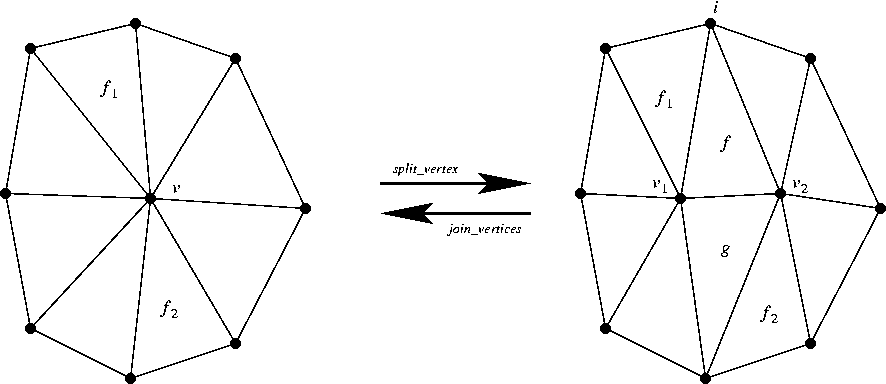## SegmentDelaunayGraphDataStructure_2

### Definition

The concept SegmentDelaunayGraphDataStructure_2 refines the concept ApolloniusGraphDataStructure_2. In addition it provides two methods for the merging of two vertices joined by an edge of the data structure, and the splitting of a vertex into two. The method that merges two vertices, called join_vertices identifies the two vertices and deletes their common two faces. The method that splits a vertex, called split_vertex introduces a new vertex that shares an edge and two faces with the old vertex (see figure below). Notice that the join_vertices and split_vertex operations are complementary, in the sense that one reverses the action of the other.Figure 31.3:  The join and split operations. Left to right: The vertex v is split into v1 and v2. The faces f and g are inserted after f1 and f2, respectively, in the counter-clockwise sense. The vertices v1, v2 and the faces f and g are returned as a boost tuple in that order. Right to left: The edge (f,i) is collapsed, and thus the vertices v1 and v2 are joined. The vertex v is returned.

We only describe the additional requirements with respect to the ApolloniusGraphDataStructure_2 concept.

### Refines

ApolloniusGraphDataStructure_2

### Modification

 Vertex_handle sdgds.join_vertices ( Face_handle f, int i) Joins the vertices that are endpoints of the edge (f,i). It returns a vertex handle to common vertex. boost::tuples::tuple sdgds.split_vertex ( Vertex_handle v, Face_handle f1, Face_handle f2) Splits the vertex v into two vertices v1 and v2. The common faces f and g of v1 and v2 are created after (in the counter-clockwise sense) the faces f1 and f2. The 4-tuple (v1,v2,f,g) is returned (see Fig. 31.7).

### Has Models

CGAL::Triangulation_data_structure_2<Vb,Fb>